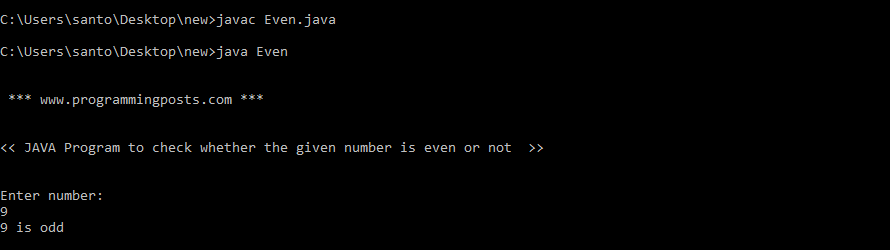# Java program to check whether the given number is even or odd

A number is said to be even if it is divisible by 2.  otherwise odd.

Let us now see an example of a java program to determine whether the given number is an odd or even number.

### Algorithm:

Step 1: Start.

Step 2:  Read n.

Step 3:  if n%2 == 0, then print n is even.

Step 4: else print n is odd.

Step 5: Stop.

### Source code:

```import java.util.Scanner;
class Even
{
public static void main(String args[])
{
System.out.println("\n \n *** www.programmingposts.com *** \n \n");
System.out.println("<<JAVA Program to check whether the given number is even or not>>\n\n");
Scanner scanner = new Scanner(System.in);
System.out.println("Enter number:");
int num = scanner.nextInt();
if(num%2 == 0)
{
System.out.println(+ num + "  is even");
}
else
{
System.out.println(+ num + " is odd");
}
}
}```

### Explanation:

In the program, integer entered by the user is stored in variable num.

Then, whether the number is perfectly divisible by 2 or not is checked using modulus operator.

If the number is perfectly divisible by 2, test expression num%2 == 0  evaluates to 1 (true) and the number is even.

However, if the test expression evaluates to 0 (false), the number is odd.

### Output:

C:\Users\santo\Desktop\new>javac Even.java

C:\Users\santo\Desktop\new>java Even

*** www.programmingposts.com ***

<< JAVA Program to check whether the given number is even or not >>

Enter number:
9
9 is odd

### Screenshot of output:Screenshot of output